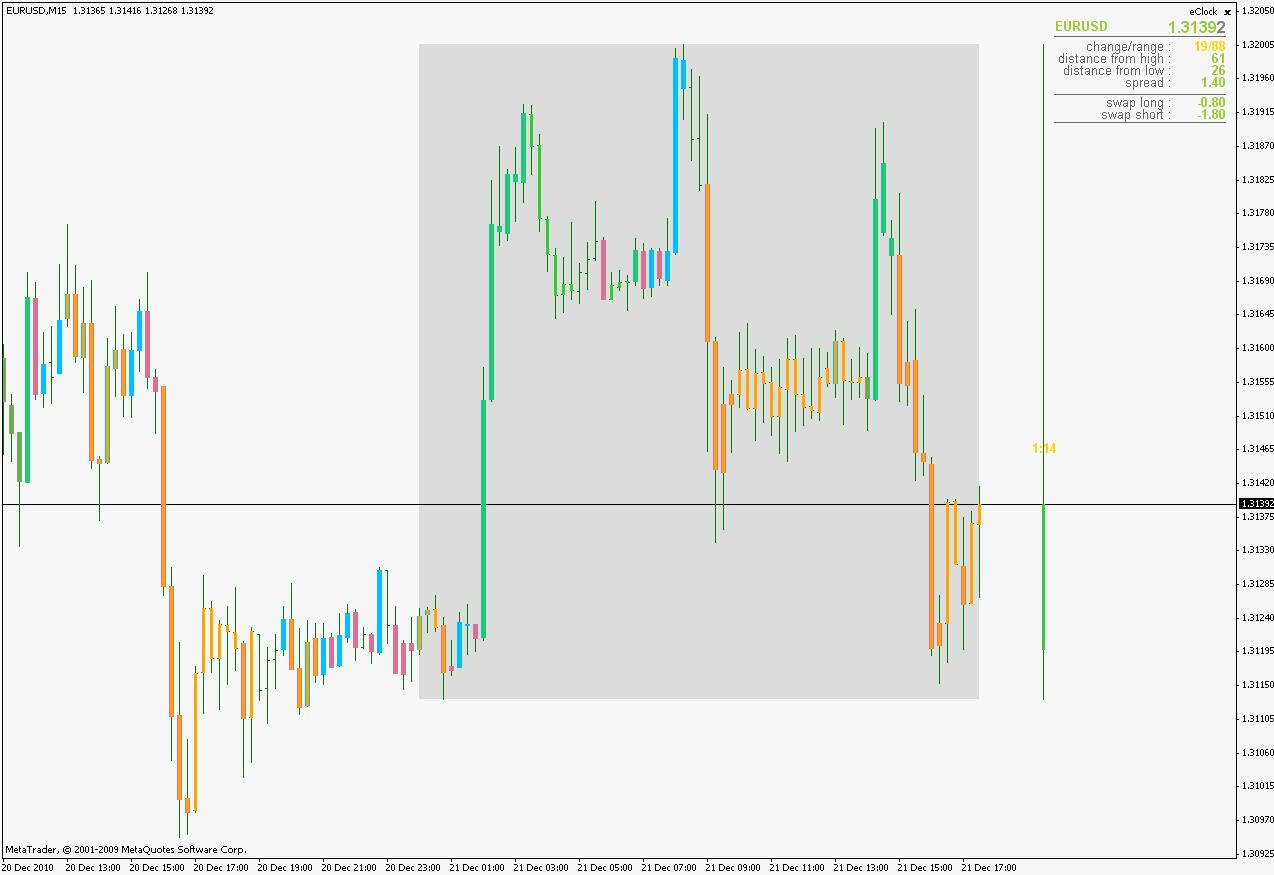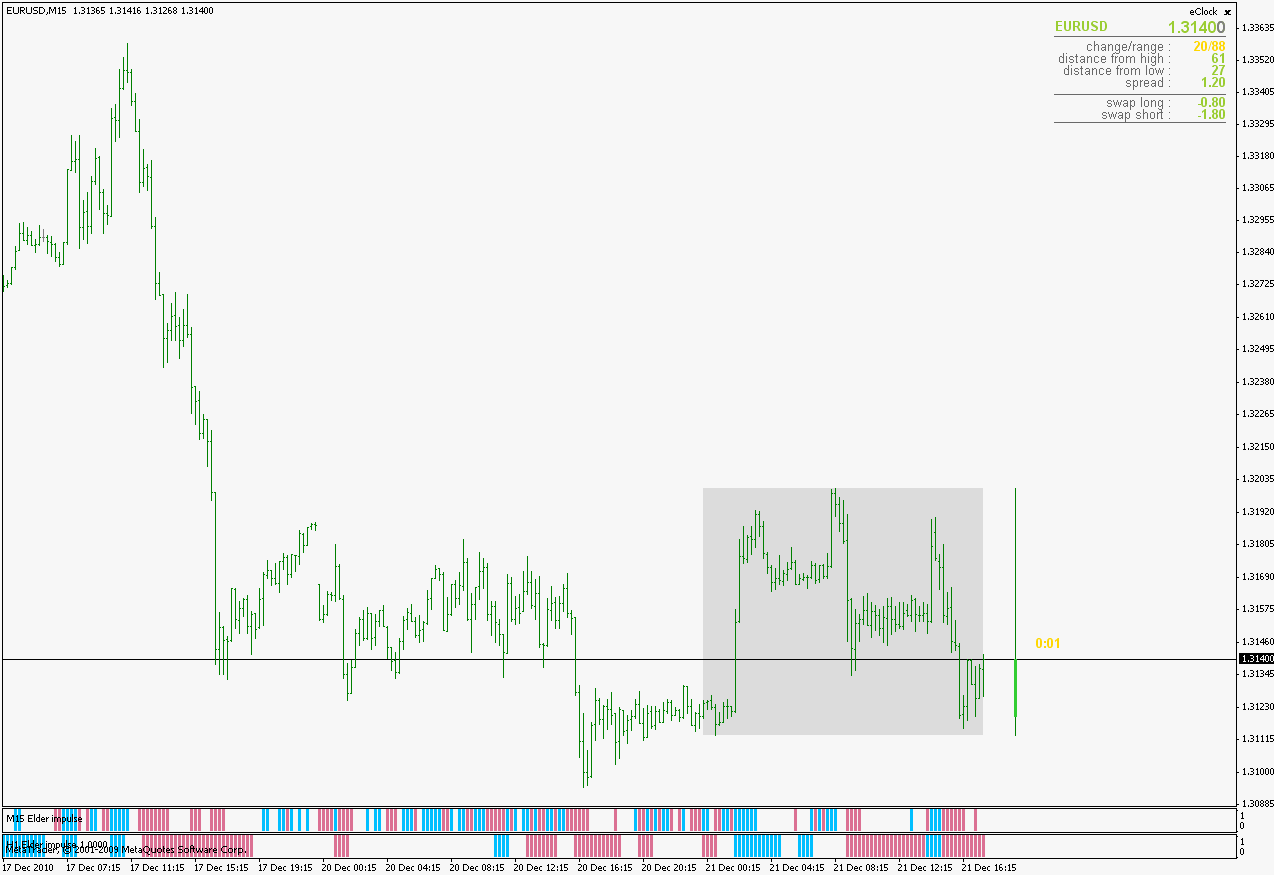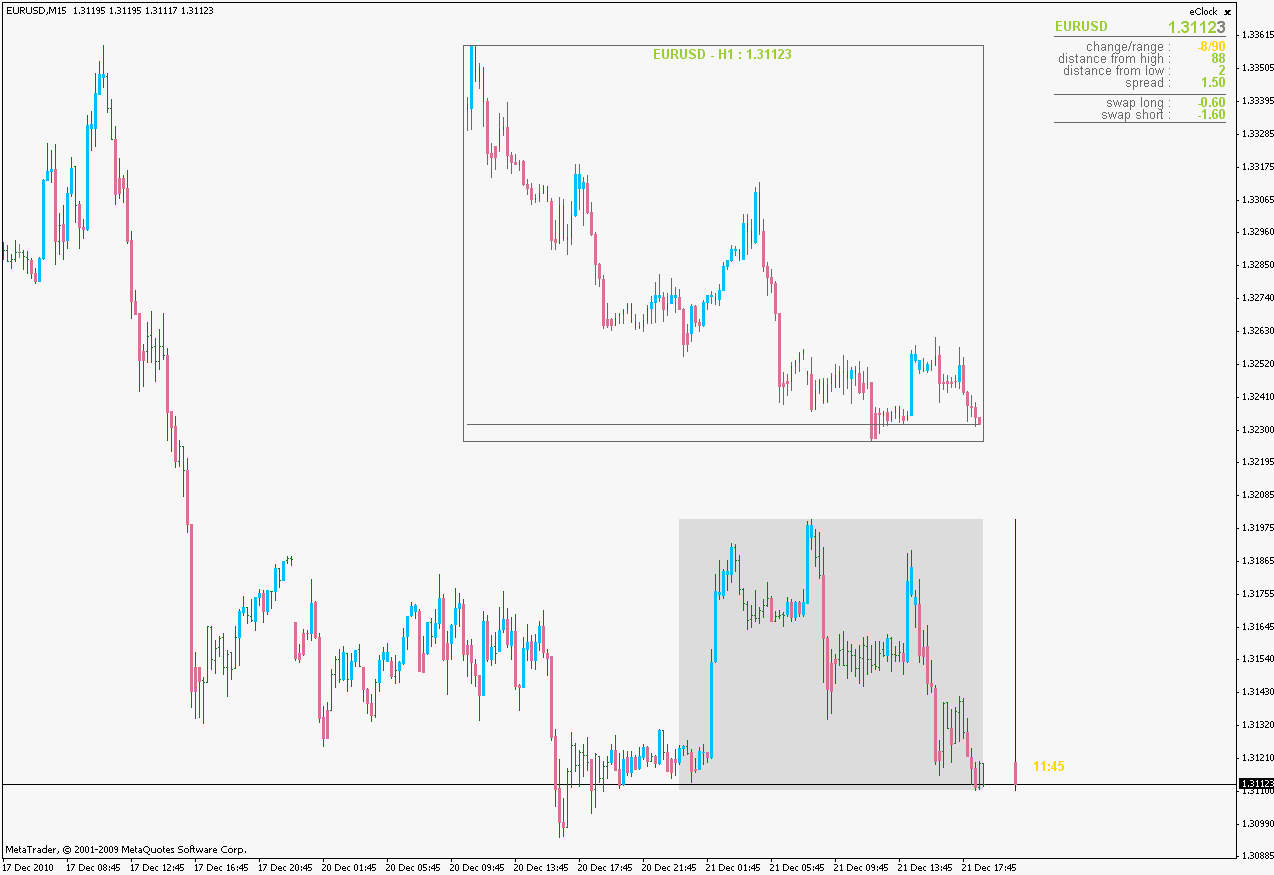Elite indicators :) - page 254519

Trading in 3 steps

Trading in 3 steps

--------------------------------------------------------------------------------

Getting in and out after 3 Candles maximum (if it goes well .. ok, if not out)

REM Programacion_11

PDS11=14

PDS21=5

PDS31=3

{PDS41=5}

PDS51=3

if Close> Average[PDS11](Close) THEN

x11=STD[PDS11](close)

ELSE

x11=(-1)*STD[PDS11](close)

ENDIF

{x21=((summation[PDS31](x11-lowest[PDS21](x11)))/summation[PDS31](highest[PDS21](x11)-lowest[PDS21](x11)))*100}

x31=x11*AverageTrueRange(close)

x41=((summation[PDS31](x31-lowest[PDS21](x31)))/summation[PDS31](highest[PDS21](x31)-lowest[PDS21](x31)))*100

{StochExSD=ExponentialAverage[PDS51](x21)}

StochExATR=ExponentialAverage[PDS51](x41)

REM Programacion_12

x1=(Close-LinearRegression(close))

if x1>x1 THEN

x2=1

ELSE

x2=0

ENDIF

if x1>x1 THEN

x3=x1-x1

ELSE

x3=0

ENDIF

if x1<x1 THEN

x4=1

ELSE

x4=0

ENDIF

if x1<x1 THEN

x5=x1-x1

ELSE

x5=0

ENDIF

s=20

x6=(summation[s](x3))*(summation[s](x2))

x7=(summation[s](x5))*(summation[s](x4))

x8=100-(100/(1+(x6/(x7+0.00001))))

REM Programacion_13

if Close< ExponentialAverage(Close) THEN

Value11=(((Low-ExponentialAverage(Low))/Close)*100)*(((AverageTrueRange(close))/Close)*100)

ELSE

Value11=(((High-ExponentialAverage(High))/Close)*100)*(((AverageTrueRange(close))/Close)*100)

ENDIF

Value22=Average(Value11)

z1=LinearRegressionSlope(StochExATR)

z2=LinearRegressionSlope(x8)

z3=LinearRegressionSlope(Value22)

y1=LinearRegression(close)

y2=AverageTrueRange(close)

y3=((y1-close)/y2)*-3

w=z1+z2+z3+y3

LineaZero=0

LineaSobrecompra=+25

LineaSobreventa=-25

uExtrem=ExponentialAverage(w)+STD(w)

lExtrem=ExponentialAverage(w)-STD(w)

RETURN w as "ABCpasos__ACC_P(ATR", LineaZero as "LineaZero", LineaSobrecompra coloured(204,0,153) as "Linea+25", LineaSobreventa coloured(204,0,153) as "Linea-25", uExtrem as "uExtrem", lExtrem as "lExtrem"

Files:519

identify the following indicators?...

May you help to identify the following indicators?... or websites

that are inside .....the Chart

Thanks

Files:
ergocci.gif 20 kb
nlitrend.gif 21 kb519

In the same Chart ElderImpul H1 , Sub Chart ElderImpul M15

Sub Chart ElderImpul M15, In the same Chart ElderImpul H1

--------------------------------------------------------------------------------

Mladen may you do

Sub Chart ElderImpul M15, In the same Chart ElderImpul H1

thanks151200

bebeshel

Here you goIt is a classical mtf and that way you can attach multiple time frames to the same chart. As an example : Elder impulse 15 minute (blue and red) and Elder impulse 1 hour (green and orange) on the same 15 minute chartBut as you can see it is hard to separate them in some situations when they overlap. So here is a histogram version of it too. That way you can attach as many as you want and they won't hide each other (same example as above) .regards

bebeshel:
Sub Chart ElderImpul M15, In the same Chart ElderImpul H1

--------------------------------------------------------------------------------

Mladen may you do

Sub Chart ElderImpul M15, In the same Chart ElderImpul H1

thanks

Updated elder impulse posted here : https://www.mql5.com/en/forum/general

Files:151200

bebeshel

In one of our conversation I told you that AverageTrueRange(close)(so average true range of a close) does not exist.

Probably different trading platforms allow different things but believe me that calculating an average true range of a close has no meaning at all. Hence, since all the variables are tied up, the last values of the calculation are questionable (deciphering what does exactly AverageTrueRange of a close return on another trading platform is yet another problem which would be required to know what exactly is calculated)

regards

bebeshel:
Trading in 3 steps

--------------------------------------------------------------------------------

Getting in and out after 3 Candles maximum (if it goes well .. ok, if not out)

REM Programacion_11

PDS11=14

PDS21=5

PDS31=3

{PDS41=5}

PDS51=3

if Close> Average[PDS11](Close) THEN

x11=STD[PDS11](close)

ELSE

x11=(-1)*STD[PDS11](close)

ENDIF

{x21=((summation[PDS31](x11-lowest[PDS21](x11)))/summation[PDS31](highest[PDS21](x11)-lowest[PDS21](x11)))*100}

x31=x11*AverageTrueRange(close)

x41=((summation[PDS31](x31-lowest[PDS21](x31)))/summation[PDS31](highest[PDS21](x31)-lowest[PDS21](x31)))*100

{StochExSD=ExponentialAverage[PDS51](x21)}

StochExATR=ExponentialAverage[PDS51](x41)

REM Programacion_12

x1=(Close-LinearRegression(close))

if x1>x1 THEN

x2=1

ELSE

x2=0

ENDIF

if x1>x1 THEN

x3=x1-x1

ELSE

x3=0

ENDIF

if x1<x1 THEN

x4=1

ELSE

x4=0

ENDIF

if x1<x1 THEN

x5=x1-x1

ELSE

x5=0

ENDIF

s=20

x6=(summation[s](x3))*(summation[s](x2))

x7=(summation[s](x5))*(summation[s](x4))

x8=100-(100/(1+(x6/(x7+0.00001))))

REM Programacion_13

if Close< ExponentialAverage(Close) THEN

Value11=(((Low-ExponentialAverage(Low))/Close)*100)*(((AverageTrueRange(close))/Close)*100)

ELSE

Value11=(((High-ExponentialAverage(High))/Close)*100)*(((AverageTrueRange(close))/Close)*100)

ENDIF

Value22=Average(Value11)

z1=LinearRegressionSlope(StochExATR)

z2=LinearRegressionSlope(x8)

z3=LinearRegressionSlope(Value22)

y1=LinearRegression(close)

y2=AverageTrueRange(close)

y3=((y1-close)/y2)*-3

w=z1+z2+z3+y3

LineaZero=0

LineaSobrecompra=+25

LineaSobreventa=-25

uExtrem=ExponentialAverage(w)+STD(w)

lExtrem=ExponentialAverage(w)-STD(w)

RETURN w as "ABCpasos__ACC_P(ATR", LineaZero as "LineaZero", LineaSobrecompra coloured(204,0,153) as "Linea+25", LineaSobreventa coloured(204,0,153) as "Linea-25", uExtrem as "uExtrem", lExtrem as "lExtrem"12

can i have SSA [gann hilo activator] of the heiken ashi .. with alerts .. please ?

cheers

Piters519

Sorry I do not fully understand me,...i am speak for, following :

Perdone creo que no me e echo entender bien ,...yo ablo de siguiente

Files:
elderh1m15.jpg 266 kb519

Es el mismo que as uzado en TTiEstochEx_v2, it is the AverageTrueRange

Thanks

Here is the message that has just been posted:

***************

bebeshel

In one of our conversation I told you that *AverageTrueRange(close) *(so average true range of a close) does not exist.

Probably different trading platforms allow different things but believe me that calculating an average true range of a close has no meaning at all hence, since all the variables are tied up, the last values of the calculation are questionable (deciphering what does exactly AverageTrueRange of a close returns on another trading platform is yet another problem which would be required to know what exactly is calculated)

regards519

En lugar de ATR usa : Close corta arriba /abajo ElderSafeZonepara las "2 signa" , de ElderTriplePantalla151200

bebeshel

Here you goFor the main chart use the "normal" Elder impulse indicator

to move the sub-chart around, use UpperHalf and FromRight parameter. Width in bars is regulated by Width parameter

regards